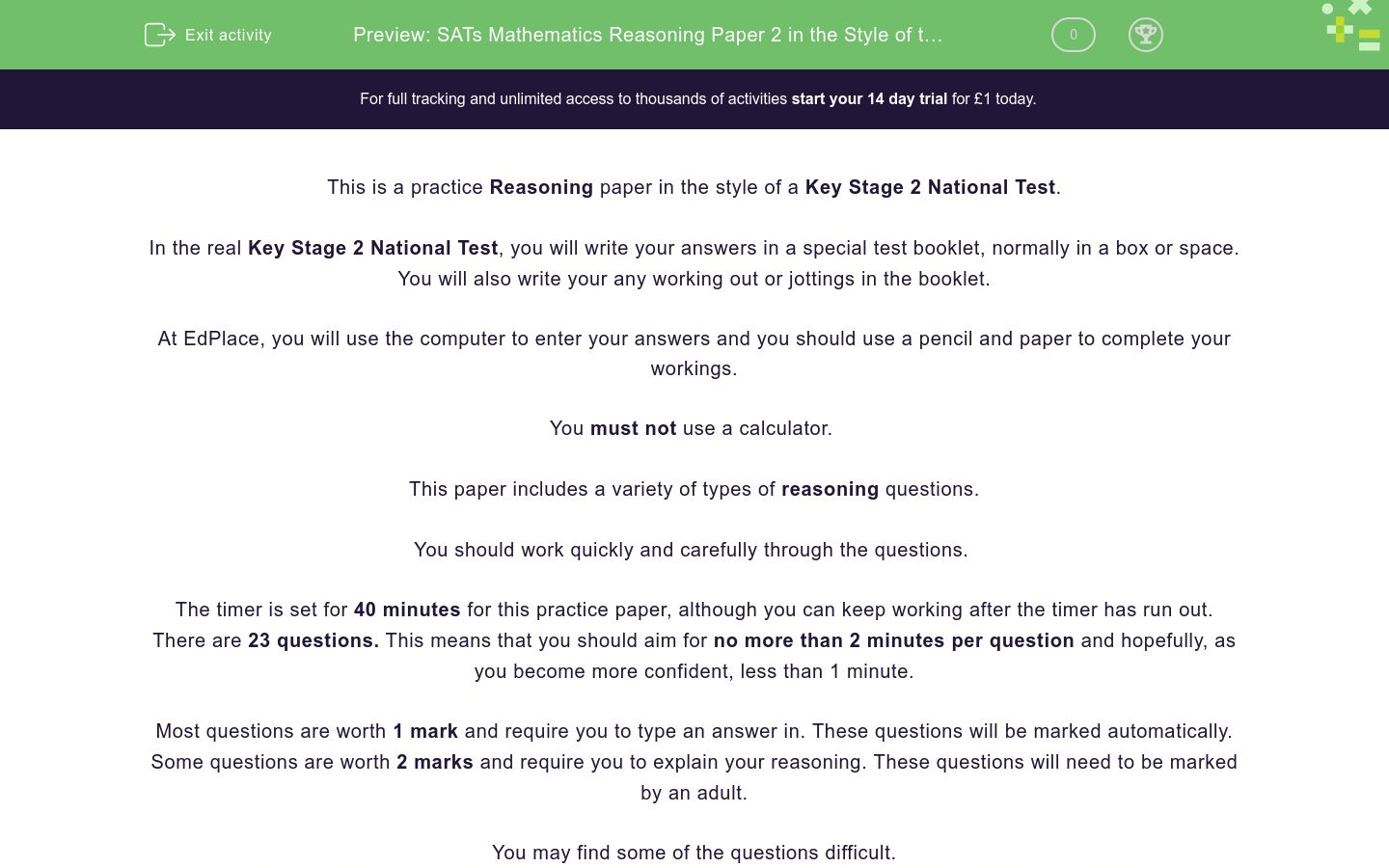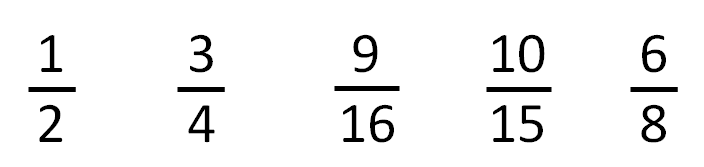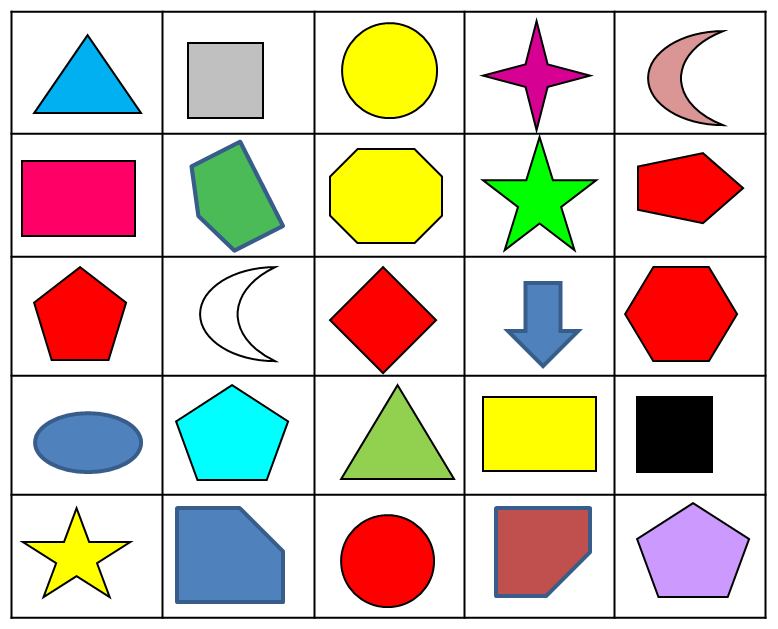# SATs Mathematics Reasoning (Paper 2) in the Style of the Key Stage 2 National Tests

In this assessment, students will be able to complete a timed Reasoning paper in the style of Key Stage 2 SATs.Key stage:  KS 2

Curriculum topic:   SATs Practice Papers

Curriculum subtopic:   Reasoning Practice Papers

Difficulty level:### QUESTION 1 of 10

This is a practice Reasoning paper in the style of a Key Stage 2 National Test.

In the real Key Stage 2 National Test, you will write your answers in a special test booklet, normally in a box or space.

You will also write your any working out or jottings in the booklet.

At EdPlace, you will use the computer to enter your answers and you should use a pencil and paper to complete your workings.

You must not use a calculator.

This paper includes a variety of types of reasoning questions.

You should work quickly and carefully through the questions.

The timer is set for 40 minutes for this practice paper, although you can keep working after the timer has run out.

There are 23 questions. This means that you should aim for no more than 2 minutes per question and hopefully, as you become more confident, less than 1 minute.

Most questions are worth 1 mark and require you to type an answer in. These questions will be marked automatically.

Some questions are worth 2 marks and require you to explain your reasoning. These questions will need to be marked by an adult.

You may find some of the questions difficult.

If you are struggling to answer a question, do not waste time on it, but move onto the next question.

Disclaimer:

We have no affiliation to the Standards and Testing Agency (STA) and these questions represent our own unique content developed by EdPlace Key Stage 2 Maths authors.

None of the content displayed here has been supplied by the STA or any other third party suppliers.

Look at these number bricks.In this question, the two lower numbers always multiply together to form the number above.

Find the numbers shown by the letters A, B and C in the arrangement of bricks below.## Column B

A
3
B
4.5
C
1.5

Look at this thermometer.

Select the temperature which is 20 degrees higher than that shown?24 °C

12 °C

16 °C

28 °C

Here is part of a number sequence.

12     17     22     27     32     ...

Select all of the numbers below that will appear in this sequence.

35

37

53

72

77

Look at this diagram.The number in the square is calculated by doubling the sum of the numbers in the circles and adding the result to the number in the square.

Calculate the missing number in the following diagram using the same rule.Match each calculation to the number that is nearest to the correct answer.

## Column B

56 × 4
300
355 + 127
400
620 ÷ 2
600
725 - 148
500
1200 ÷ 3
200

Raj sees this sign at the local store.How much does one can cost in pence?

## Column B

56 × 4
300
355 + 127
400
620 ÷ 2
600
725 - 148
500
1200 ÷ 3
200

Here are five fractions.Select two which are equivalent.

1/2

3/4

9/16

10/15

6/8

Select the largest.

4/9

1/3

30%

0.4

Increase 45 by 20%.

An equilateral triangle is shown inside a square.The perimeter of the triangle is 42 cm.

Calculate the perimeter of the square.

Find the area of the shaded shape.

Each square is 1 cm by 1 cm.Ann is half as tall as her father.

Her father is 10 cm taller than her mother.

Ann's mother is 172 cm tall.

How many centimetres tall is Ann?

What percentage of this selection of shapes are not pentagons?Four children grow sunflowers in their gardens.

This bar chart shows how high their sunflowers reached in the summer.What is the height of Aisha's sunflower to the nearest metre?

Find a prime number between 90 and 100.

89

92

93

97

91

A rectangular window is 1½ metres high.

Its width is 25 cm longer than its height.

How wide is the window in metres?

Here are five calculations.

Select the calculations whose answers are less than 340.

3.35 × 100

842 ÷ 2

¼ of 1200

124 + 134 + 82

11 × 30

Look at this net of a cube.Which face will be opposite the blue face when it is folded to form a cube?

A

B

C

D

E

In a bag, 21 counters are red and the rest are blue.

A quarter of the counters are blue.

Altogether, how many counters are there in the bag?

A bag contains coloured counters.

There are 4 red, 5 yellow and 6 blue.

Aisha says "One-third of the counters are yellow."

Is Aisha correct?

Tracey and Aimen find part of an incomplete sequence.Aimen and Tracey both make a list of numbers which they think could appear in this sequence:

 Aimen Tracey 0, -15, -30, 95, 105, 120, 45 45,  90,  105, 150, 135, -75

Whose list is correct?

The diagram shows two similar parallelograms.Calculate the perimeter of rectangle B in mm.

A rectangle and its diagonal are shown below.Tom estimates that angle x is 55°.

Sidney estimates that angle x is 62°.

Whose answer is closest to the actual size of angle x?

• Question 1

Look at these number bricks.In this question, the two lower numbers always multiply together to form the number above.

Find the numbers shown by the letters A, B and C in the arrangement of bricks below.## Column B

A
1.5
B
3
C
4.5
EDDIE SAYS
The answers are as follows: A = 1.5 ÷ 1 = 1.5 B = 1.5 × 2 = 3 C = 1.5 × 3 = 4.5 Remember that 1.5 just means one and a half.
• Question 2

Look at this thermometer.

Select the temperature which is 20 degrees higher than that shown?12 °C
EDDIE SAYS
The correct answer is 12. Each small division is worth 2 °C. The temperature shown is -8 °C. -8 + 20 = 12
• Question 3

Here is part of a number sequence.

12     17     22     27     32     ...

Select all of the numbers below that will appear in this sequence.

37
72
77
EDDIE SAYS
The correct numbers are 37, 72 and 77. The numbers go up by 5 each time in this sequence. Well spotted if you also noticed that the ones digit in each number is always either 2 or 7!
• Question 4

Look at this diagram.The number in the square is calculated by doubling the sum of the numbers in the circles and adding the result to the number in the square.

Calculate the missing number in the following diagram using the same rule.27
EDDIE SAYS
The missing number in the second diagram will be 27. First, add the numbers in the circles. 7 + 5 = 12 Double this. 12 × 2 = 24 Add the number in the square. 24 + 3 = 27
• Question 5

Match each calculation to the number that is nearest to the correct answer.

## Column B

56 × 4
200
355 + 127
500
620 ÷ 2
300
725 - 148
600
1200 ÷ 3
400
EDDIE SAYS
The answers are as follows: 56 × 4 = 224 ≅ 200 355 + 127 = 482 ≅ 500 620 ÷ 2 = 310 ≅ 300 725 - 148 = 577 ≅ 600 1200 ÷ 3 = 400 ≅ means 'approximately equal to'.
• Question 6

Raj sees this sign at the local store.How much does one can cost in pence?

EDDIE SAYS
The answer is 47 pence. Short division or 'bus stop' method is useful for these type of questions. 282 ÷ 6 = 47 6 does not fit into 2, so we carry over the 2. 6 fits into 28 four times with 4 to carry over. 6 fits into 42 exactly seven times.
• Question 7

Here are five fractions.Select two which are equivalent.

3/4
6/8
EDDIE SAYS
The correct answers are 3/4 and 6/8. 3/4 is equivalent to 6/8 because if you double 3 you get 6, and if you double 4 you get 8. Top tip: When making equivalent fractions, always do the same thing to the numerator and denominator.
• Question 8

Select the largest.

4/9
EDDIE SAYS
The correct answer is 4/9. Check the decimals, fractions and percentages to compare them: 30% = 0.3 so this is smaller than 0.4. 1/3 = 3/9 so this is smaller than 4/9. 0.4 = 4/10 so this is smaller than 4/9.
• Question 9

Increase 45 by 20%.

54
EDDIE SAYS
The correct answer is 54. There are two simple ways you can solve this question. Method one 10% of 45 = 4.5 20% of 45 = 9 45 + 9 = 54 Method two We know that 20% = 1/5 1/5 of 45 is 9 45 + 9 = 54
• Question 10

An equilateral triangle is shown inside a square.The perimeter of the triangle is 42 cm.

Calculate the perimeter of the square.

56
EDDIE SAYS
The answer is 56 cm. We're told that the triangle is equilateral, which means it has equal sides. Each side of the triangle is 14 cm. 42 ÷ 3 = 14 So, the sides of the square are also each 14 cm. The perimeter of the square is 56 cm. 4 × 14 = 56
• Question 11

Find the area of the shaded shape.

Each square is 1 cm by 1 cm.51
EDDIE SAYS
The answer is 51 cm² There are 49 full squares. There are also 4 half squares which add together to make 2 whole squares. 49 + 2 = 51
• Question 12

Ann is half as tall as her father.

Her father is 10 cm taller than her mother.

Ann's mother is 172 cm tall.

How many centimetres tall is Ann?

91
EDDIE SAYS
The answer is 91 cm. Ann's father is 10 cm taller than her mother. 172 + 10 = 182 Ann is half as tall as her father. 182 ÷ 2 = 91
• Question 13

What percentage of this selection of shapes are not pentagons?72
EDDIE SAYS
The answer is 72%. There are 25 shapes, of which 7 are pentagons (5 sided shapes). This means there are 18 shapes which are not pentagons. 18/25 = 72/100 = 72%
• Question 14

Four children grow sunflowers in their gardens.

This bar chart shows how high their sunflowers reached in the summer.What is the height of Aisha's sunflower to the nearest metre?

2
EDDIE SAYS
The answer is 2 m. Aisha's sunflower is just over 2 m but less than 2.5 m, so nearer to 2 m than to 3 m.
• Question 15

Find a prime number between 90 and 100.

91
EDDIE SAYS
The only prime number between 90 and 100 is 97. Remember that prime numbers can only be divided by themselves and one. 89 is prime but is not between 90 and 100. 92 is even so not prime. 93 is divisible by 3 so not prime. 97 is prime as its only factors are 1 and 97. 91 is divisible by 7 so not prime.
• Question 16

A rectangular window is 1½ metres high.

Its width is 25 cm longer than its height.

How wide is the window in metres?

1.75
EDDIE SAYS
The window is 175 cm wide. 1½ metres = 150 cm 150 + 25 = 175 175 cm is equal to 1.75m.
• Question 17

Here are five calculations.

Select the calculations whose answers are less than 340.

3.35 × 100
¼ of 1200
11 × 30
EDDIE SAYS
The correct answers are: 3.35 × 100, ¼ of 1200 and 11 × 30. 3.35 × 100 = 335 This is less than 340. 842 ÷ 2 = 421 This is greater than 340. ¼ of 1200 = 1200 ÷ 4 = 300 This is less than 340. 124 + 134 + 82 = 340 This is equal to 340. 11 × 30 = 330 This is less than 340.
• Question 18

Look at this net of a cube.Which face will be opposite the blue face when it is folded to form a cube?

A
EDDIE SAYS
The correct answer is A Opposite faces on a cube are always separated by exactly one face. Imagine the blue face as the base. B will be the back, C will be the right face and A will be on top.
• Question 19

In a bag, 21 counters are red and the rest are blue.

A quarter of the counters are blue.

Altogether, how many counters are there in the bag?

28
EDDIE SAYS
The answer is 28. 21 of the counters are red and one-quarter of the counters are blue. This means 21 counters represent three-quarters of the counters so one quarter is 7. 21 ÷ 3 = 7 Altogether, the counters represent one whole or four quarters. 7 × 4 - 28
• Question 20

A bag contains coloured counters.

There are 4 red, 5 yellow and 6 blue.

Aisha says "One-third of the counters are yellow."

Is Aisha correct?

EDDIE SAYS
Aisha is correct. There are 15 counters in the bag. 4 + 5 + 6 = 15 One-third of 15 is 5. 15 ÷ 3 =5
• Question 21

Tracey and Aimen find part of an incomplete sequence.Aimen and Tracey both make a list of numbers which they think could appear in this sequence:

 Aimen Tracey 0, -15, -30, 95, 105, 120, 45 45,  90,  105, 150, 135, -75

Whose list is correct?

EDDIE SAYS
Tracey's list is correct. The gaps in the sequence are exactly 15 each time so the numbers in Tracey's list could fill in the gaps in the sequence and could also appear before and after the part of the sequence shown. Aimen's list contains the number 95, which would not appear in the sequence.
• Question 22

The diagram shows two similar parallelograms.Calculate the perimeter of rectangle B in mm.

EDDIE SAYS
The perimeter of shape B is 330 mm. We can see that the measurement of 4 cm has increased to 6 cm. This is because a scale factor of 1.5 has been used. 4 × 1.5 = 6 In similar shapes, we need to apply the same scale factor to all dimensions. 7 × 1.5 = 10.5 To find the perimeter, add together all the sides. 6 + 6 + 10.5 + 10.5 = 33 Finally, you'll need to multiply by ten to convert cm to mm. 33 × 10 = 330
• Question 23

A rectangle and its diagonal are shown below.Tom estimates that angle x is 55°.

Sidney estimates that angle x is 62°.

Whose answer is closest to the actual size of angle x?

EDDIE SAYS
Tom's estimate is closest. The angles in a triangle add to 180°. Right angles (90°) are marked with a square angle marker. The missing angle is 58°. 180 - 90 - 32 = 90 - 32 = 58 Tom's estimate is 3 less than 58. Sidney's estimate is 4 more than 58.
Try it ---- OR ----

Sign up for a £1 trial so you can track and measure your child's progress on this activity.

### What is EdPlace?

We're your National Curriculum aligned online education content provider helping each child succeed in English, maths and science from year 1 to GCSE. With an EdPlace account you’ll be able to track and measure progress, helping each child achieve their best. We build confidence and attainment by personalising each child’s learning at a level that suits them.

Get started